# Arithmetic Reasoning

• 13 Questions
• 54 Problems
• 36 Flash Cards

### Fundamentals

##### Number Properties
###### Integers

An integer is any whole number, including zero. An integer can be either positive or negative. Examples include -77, -1, 0, 55, 119.

###### Rational Numbers

A rational number (or fraction) is represented as a ratio between two integers, a and b, and has the form $${a \over b}$$ where a is the numerator and b is the denominator. An improper fraction ($${5 \over 3}$$) has a numerator with a greater absolute value than the denominator and can be converted into a mixed number ($$1 {2 \over 3}$$) which has a whole number part and a fractional part.

###### Absolute Value

The absolute value is the positive magnitude of a particular number or variable and is indicated by two vertical lines: $$\left|-5\right| = 5$$. In the case of a variable absolute value ($$\left|a\right| = 5$$) the value of a can be either positive or negative (a = -5 or a = 5).

###### Factors & Multiples

A factor is a positive integer that divides evenly into a given number. The factors of 8 are 1, 2, 4, and 8. A multiple is a number that is the product of that number and an integer. The multiples of 8 are 0, 8, 16, 24, ...

###### Greatest Common Factor

The greatest common factor (GCF) is the greatest factor that divides two integers.

###### Least Common Multiple

The least common multiple (LCM) is the smallest positive integer that is a multiple of two or more integers.

###### Prime Number

A prime number is an integer greater than 1 that has no factors other than 1 and itself. Examples of prime numbers include 2, 3, 5, 7, and 11.

##### Operations on Fractions
###### Simplifying Fractions

Fractions are generally presented with the numerator and denominator as small as is possible meaning there is no number, except one, that can be divided evenly into both the numerator and the denominator. To reduce a fraction to lowest terms, divide the numerator and denominator by their greatest common factor (GCF).

Fractions must share a common denominator in order to be added or subtracted. The common denominator is the least common multiple of all the denominators.

###### Multiplying & Dividing Fractions

To multiply fractions, multiply the numerators together and then multiply the denominators together. To divide fractions, invert the second fraction (get the reciprocal) and multiply it by the first.

##### Operations on Exponents
###### Defining Exponents

An exponent (cbe) consists of coefficient (c) and a base (b) raised to a power (e). The exponent indicates the number of times that the base is multiplied by itself. A base with an exponent of 1 equals the base (b1 = b) and a base with an exponent of 0 equals 1 ( (b0 = 1).

To add or subtract terms with exponents, both the base and the exponent must be the same. If the base and the exponent are the same, add or subtract the coefficients and retain the base and exponent. For example, 3x2 + 2x2 = 5x2 and 3x2 - 2x2 = x2 but x2 + x4 and x4 - x2 cannot be combined.

###### Multiplying & Dividing Exponents

To multiply terms with the same base, multiply the coefficients and add the exponents. To divide terms with the same base, divide the coefficients and subtract the exponents. For example, 3x2 x 2x2 = 6x4 and $${8x^5 \over 4x^2}$$ = 2x(5-2) = 2x3.

###### Exponent to a Power

To raise a term with an exponent to another exponent, retain the base and multiply the exponents: (x2)3 = x(2x3) = x6

###### Negative Exponent

A negative exponent indicates the number of times that the base is divided by itself. To convert a negative exponent to a positive exponent, calculate the positive exponent then take the reciprocal: $$b^{-e} = { 1 \over b^e }$$. For example, $$3^{-2} = {1 \over 3^2} = {1 \over 9}$$

Radicals (or roots) are the opposite operation of applying exponents. With exponents, you're multiplying a base by itself some number of times while with roots you're dividing the base by itself some number of times. A radical term looks like $$\sqrt[d]{r}$$ and consists of a radicand (r) and a degree (d). The degree is the number of times the radicand is divided by itself. If no degree is specified, the degree defaults to 2 (a square root).

The radicand of a simplified radical has no perfect square factors. A perfect square is the product of a number multiplied by itself (squared). To simplify a radical, factor out the perfect squares by recognizing that $$\sqrt{a^2} = a$$. For example, $$\sqrt{64} = \sqrt{16 \times 4} = \sqrt{4^2 \times 2^2} = 4 \times 2 = 8$$.

To add or subtract radicals, the degree and radicand must be the same. For example, $$2\sqrt{3} + 3\sqrt{3} = 5\sqrt{3}$$ but $$2\sqrt{2} + 2\sqrt{3}$$ cannot be added because they have different radicands.

To multiply or divide radicals, multiply or divide the coefficients and radicands separately: $$x\sqrt{a} \times y\sqrt{b} = xy\sqrt{ab}$$ and $${x\sqrt{a} \over y\sqrt{b}} = {x \over y}\sqrt{a \over b}$$

###### Square Root of a Fraction

To take the square root of a fraction, break the fraction into two separate roots then calculate the square root of the numerator and denominator separately. For example, $$\sqrt{9 \over 16}$$ = $${\sqrt{9}} \over {\sqrt{16}}$$ = $${3 \over 4}$$

##### Miscellaneous
###### Scientific Notation

Scientific notation is a method of writing very small or very large numbers. The first part will be a number between one and ten (typically a decimal) and the second part will be a power of 10. For example, 98,760 in scientific notation is 9.876 x 104 with the 4 indicating the number of places the decimal point was moved to the left. A power of 10 with a negative exponent indicates that the decimal point was moved to the right. For example, 0.0123 in scientific notation is 1.23 x 10-2.

###### Factorials

A factorial has the form n! and is the product of the integer (n) and all the positive integers below it. For example, 5! = 5 x 4 x 3 x 2 x 1 = 120.

### Applications

##### Order of Operations
###### PEMDAS

Arithmetic operations must be performed in the following specific order:

1. Parentheses
2. Exponents
3. Multiplication and Division (from L to R)
4. Addition and Subtraction (from L to R)

The acronym PEMDAS can help remind you of the order.

###### Distributive Property - Multiplication

The distributive property for multiplication helps in solving expressions like a(b + c). It specifies that the result of multiplying one number by the sum or difference of two numbers can be obtained by multiplying each number individually and then totaling the results: a(b + c) = ab + ac. For example, 4(10-5) = (4 x 10) - (4 x 5) = 40 - 20 = 20.

###### Distributive Property - Division

The distributive property for division helps in solving expressions like $${b + c \over a}$$. It specifies that the result of dividing a fraction with multiple terms in the numerator and one term in the denominator can be obtained by dividing each term individually and then totaling the results: $${b + c \over a} = {b \over a} + {c \over a}$$. For example, $${a^3 + 6a^2 \over a^2} = {a^3 \over a^2} + {6a^2 \over a^2} = a + 6$$.

###### Commutative Property

The commutative property states that, when adding or multiplying numbers, the order in which they're added or multiplied does not matter. For example, 3 + 4 and 4 + 3 give the same result, as do 3 x 4 and 4 x 3.

##### Ratios
###### Ratios

Ratios relate one quantity to another and are presented using a colon or as a fraction. For example, 2:3 or $${2 \over 3}$$ would be the ratio of red to green marbles if a jar contained two red marbles for every three green marbles.

###### Proportions

A proportion is a statement that two ratios are equal: a:b = c:d, $${a \over b} = {c \over d}$$. To solve proportions with a variable term, cross-multiply: $${a \over 8} = {3 \over 6}$$, 6a = 24, a = 4.

###### Rates

A rate is a ratio that compares two related quantities. Common rates are speed = $${distance \over time}$$, flow = $${amount \over time}$$, and defect = $${errors \over units}$$.

###### Percentages

Percentages are ratios of an amount compared to 100. The percent change of an old to new value is equal to 100% x $${ new - old \over old }$$.

##### Statistics
###### Averages

The average (or mean) of a group of terms is the sum of the terms divided by the number of terms. Average = $${a_1 + a_2 + ... + a_n \over n}$$

###### Sequence

A sequence is a group of ordered numbers. An arithmetic sequence is a sequence in which each successive number is equal to the number before it plus some constant number.

###### Probability

Probability is the numerical likelihood that a specific outcome will occur. Probability = $${ \text{outcomes of interest} \over \text{possible outcomes}}$$. To find the probability that two events will occur, find the probability of each and multiply them together.

##### Word Problems
###### Practice

Many of the arithmetic reasoning problems on the ASVAB will be in the form of word problems that will test not only the concepts in this study guide but those in Math Knowledge as well. Practice these word problems to get comfortable with translating the text into math equations and then solving those equations.

# Automotive Information

• 103 Questions
• 103 Flash Cards

### Engine Fundamentals

##### Components
###### Internal Combustion

Combustion is the burning of an air-fuel mixture to provide energy. It requires the presence of air, fuel, and a heat source to ignite the air-fuel mixture. In the internal combustion engine that powers automobiles and trucks the combustion happens inside the engine utilzing a fuel like gasoline, diesel fuel, or natural gas.

###### Engine Block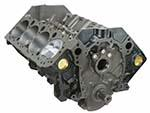The engine (or cylinder) block is the large casing that contains the cylinders and many of the internal components of the engine.

###### Cylinders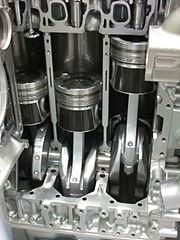Cylinders act as a guide for the pistons that translate the heat energy of combustion into the mechanical energy necessary to move a vehicle. Piston rings seal the piston to the cylinder to contain combustion gases and also regulate the oil distribution between the piston and cylinder wall. A cylinder head closes in the top of the cylinder forming the combustion chamber which is sealed by a head gasket (head). The head provides space for air and fuel intake valves, exhaust valves, and mounts for spark plugs and fuel injectors.

###### Combustion Chamber

The combustion chamber is located in the cylinder head and contains the combustion of the air-fuel mixture. This mixture is delivered by an intake valve and the waste gases from combustion are removed from the combustion chamber by the exhaust valve.

###### Connecting Rod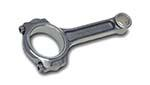A connecting rod employs a wrist pin to link each piston to the engine's crankshaft.

###### Crankshaft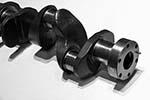The crankshaft converts the reciprocating motion of the piston into the rotational motion that's used to power the vehicle and its components.

###### Camshaft

The camshaft is linked to the crankshaft through a timing belt and regulates the opening and closing of the intake and exhaust valves in each cylinder in time with the motion of the piston. An engine designated OverHead Camshaft (OHC) locates the camshaft in the cylinder head. An engine with Double OverHead Camshaft (DOHC) has two camshafts, one to regulate the intake valves and one to regulate the exhaust valves.

###### Cylinder Arrangement

Cylinder number and arrangement depends on the purpose of the engine. Smaller (four and six cylinder) engines in front-wheel drive vehicles often use an inline design which orients cylinders vertically over the crankshaft and aligns them in a row. Other common orientations are a horizontal/opposed design which places cylinders flat facing each other with the crankshaft between them and a V-type design common in six and eight cylinder engines that features one cylinder head per block of cylinders oriented at a 60 to 90 degree angle to each other with the crankshaft at the bottom of the V.

##### Stroke Cycle
###### Intake Stroke

The four-stroke piston cycle of internal combustion engines starts with the piston at top of the cylinder head (top dead center or TDC) during the intake stroke. The piston moves downward in the cylinder creating a vacuum that pulls an air-fuel mix into the combustion chamber through the now open intake valve.

###### Compression Stroke

During the compression stroke, both intake and exhaust valves are closed as the piston begins moving back up from the bottom of the cylinder (bottom dead center or BDC). This compresses the air-fuel mixture in the combustion chamber which also makes it hotter.

###### Power Stroke

During the power stroke, just before the piston reaches top dead center, the spark plug fires and ignites the compressed air-fuel mixture. The resulting expansion due to combustion pushes the piston back down the cylinder toward bottom dead center.

###### Exhaust Stroke

During the exhaust stroke, just before the piston reaches bottom dead center the exhaust valve opens. The resulting gases from combustion are then pushed out through the exhaust valve as the piston travels up the cylinder to top dead center, completing stroke four of the four-stroke piston cycle.

##### Operation
###### Firing Order

The stroke cycle of an engine is governed by the crankshaft which serves to regulate the firing order of the cylinders. All cylinders are not on the same stroke at the same time and correct firing order is important to balance engine operation and minimize vibrations. A common firing order for four-cylinder engines is 1-3-4-2 which indicates that cylinders 1 and 3 fire (power stroke)together and cylinders 4 and 2 fire together.

###### Air-Fuel Mixture

The stoichiometric ratio defines the proper ratio of air to fuel necessary so that an engine burns all fuel with no excess air. For gasoline fuel, the stoichiometric ratio is about 14.7:1 or for every one gram of fuel, 14.7 grams of air are required. Too much air results in a lean air-fuel mixture that burns more slowly and hotter while too much fuel results in a rich mixture that burns quicker and cooler.

###### Ignition Timing

Ignition timing defines the point in time at the end of the compression stroke that the spark plug fires. Measured in number of degrees before top dead center (BTDC), the exact point that the spark plugs initiate combustion varies depending on the speed of the engine. The timing is advanced (the spark plugs fire a few more degrees BTDC) when the engine is running faster and retarded when it's running slower.

###### Combustion

Normal combustion in an engine is initiated by a spark plug and results in the complete burning of the air-fuel mixture. If combustion is initiated by a source other than the spark plug, by a hot spot in the cylinder or combustion chamber for example, pre-ignition results. Detonation results if the air-fuel mixture explodes instead of burning. Detonation can cause extremes in pressure in the combustion chamber leading to engine damage.

### Engine Systems

##### Cooling System
###### Coolant

Modern car engines are cooled by liquid which circulates through the engine block and cylinder heads absorbing excess heat. This liquid is made up of half water and half antifreeze (commonly, ethylene glycol) which both keeps the water from freezing at low temperatures and raises its boiling point making heat transfer more efficient.

###### Water Pump

The water pump is driven by a belt connected to the crankshaft and ensures that coolant moves through the engine and radiator.

###### Water Jacket

A water jacket is a coolant-filled casing that allows heat transfer from the engine block and cylinder heads to the liquid coolant.

###### Thermostat

The thermostat controls coolant (and, through it, engine) temperature by regulating the flow of coolant through the radiator. A bypass tube allows coolant to bypass the radiator and flow back into the water pump when its temperature is low enough that the thermostat is closed.

The radiator is responsible for tranferring heat from the coolant to the outside air. Radiator hoses transfer coolant to and from the engine to the radiator and a radiator cap maintains pressure in the cooling system to increase the boiling point of the coolant mixture and thus allow it to absorb more heat.

##### Lubrication System
###### Purpose

The lubrication system lubricates engine components by putting an oil film between them to reduce friction and smooth engine operation, cools by absorbing heat from engine parts, seals the pistons and cylinders to contain combustion, cleans contaminants, and quiets engine noise.

###### Oil Viscosity

The primary component of the lubrication system is engine oil. Engines require oil blends with different thickness (viscosity) and additives depending on their operating conditions. Viscosity is rated using the format XW-XX with the number preceding the W (winter) rating the oil’s viscosity at 0 ℉ (-17.8 ℃) and the XX indicating viscosity at 100 ℃.

###### Oil Pump

The oil pump is driven by the camshaft and is responsible for pumping oil through the oil galleries (passages) that run throughout the engine. It also contains the oil filter and a pressure relief valve which prevents excessive pressure from building up in the lubrication system.

###### Oil Pan

The oil pan contains the engine oil reservoir of from four to six quarts of oil and feeds the oil pump through the oil pickup tube. An oil strainer floats at the top of the oil in the oil pan and screens debris from the oil before feeding it to the oil pump.

##### Fuel System
###### Fuel Injector

The fuel injector sprays fuel into the air stream that's being fed into the cylinder head via the intake valve. The timing and amount of fuel are regulated by the powertrain control module (PCM) which is the main computer that controls engine and transmission functions.

###### Electric Fuel Pump

The electric fuel pump feeds pressurized fuel through a fuel filter to the fuel injectors via the fuel rail manifold. The fuel rail contains the fuel pressure regulator which ensures that the fuel injectors receive fuel at a consistent and known rate. Excess fuel bled off by the pressure regulator returns to the fuel tank through the fuel return line.

###### Intake Manifold

The intake manifold distributes outside air to the intake ports on the cylinder heads. The intake air filter removes any airborne contaminants before the air enters the engine.

##### Ignition System
###### Battery

The battery supplies the power necessary to start the engine when the ignition switch is is turned on.

###### Ignition Coil

The ignition coil is a high-voltage transformer made up of two coils of wire. The primary coil winding is the low-voltage winding and has relatively few turns of heavy wire. The secondary coil winding is the high-voltage winding that surrounds the primary and is made up of thousands of turns of fine wire. Current flows from the battery through the primary coil winding which creates a changing magnetic field inside the secondary coil. This induces a very high-voltage current in the secondary coil which it feeds to the distributor.

###### Distributor

The distributor is driven by the engine's camshaft and is responsible for timing the spark and distributing it to the correct cylinder. The distributor cap contains a rotor that connects the ignition coil (and its high voltage) to the proper cylinder at the proper point in the stroke cycle.

###### Spark Plugs

Spark plugs receive current from the distributor and use it to spark combustion in the combustion chamber of a cylinder.

##### Exhaust System
###### Exhaust Manifolds

The cast iron exhaust manifolds collect engine exhaust gas from multiple cylinder exhaust valves and deliver it to the exhaust pipe. Exhaust manifolds can be generic or specially tuned (header pipes) to the engine. Header pipes deliver higher performance but are more expensive and less durable.

###### Catalytic Converter

The catalytic converter converts pollutants in exhaust gas into less pollutant substances like carbon dioxide and water.

###### Muffler

The muffler follows the catalytic converter and absorbs sound to help quiet load exhaust. It is followed by the exhaust pipe which is the final exit point for exhaust gas from the vehicle.

### Electrical and Computer Systems

##### Electrical System Components
###### Battery

The lead-acid battery is the core of the electrical system, providing current to the ignition system to start the engine as well as delivering supplemental current when the alternator can't handle high electrical system loads and acting as an electrical reservoir for excessive current.

###### Solenoid

The cylindrical solenoid is a relay that safely connects the high amperage battery to the starter motor when the ignition key is turned. This current then allows the engine to turn at a high enough speed to start.

###### Alternator

Once the engine is running, the alternator provides electrical current to recharge the battery and power the electrical system. The alternator is driven by the engine's crankshaft and produces alternating current (AC) which is then fed through a rectifier bridge to convert it to the direct current (DC) required by the electrical system. A voltage regulator controls the output of the alternator to maintain a consistent voltage (approx. 14.5 volts) in the electrical system regardless of load.

###### Lighting

The lighting system consists of interior lights, instrument panel lighting, headlights, and taillights. Like household electrical circuits, the vehicle's lighting system is protected from current spikes by fuses and circuit breakers.

##### Computer System Components
###### Sensors

Sensors provide the data necessary for the vehicle's computer to make decisions and monitor everything from simple vehicle information like tire pressure to complexities like the chemical content of an engine's exhaust.

###### Powertrain Control Module

The main computer or powertrain control module (PCM) uses pre-programmed software to analyze the input received from sensors and produce output signals to adjust vehicle performance and operation. (Engine control unit (ECU) is another name for the PCM.)

###### Actuators

Actuators receive signals from the powertrain control module and carry out adjustments needed based on the data the PCM received from the sensors.

### Operational Systems

##### Drive Train
###### Transmission

The transmission provides the appropriate power to vehicle wheels to maintain a given speed. The engine and the transmission have to be disconnected to shift gears and a manual transmission requires the driver to manually manage this disconnection (using a clutch) and to manually shift gears. An automatic transmission is essentially an automatic gear shifter and handles this process without driver input.

###### Transaxle

A differential is designed to drive a pair of wheels while allowing them to rotate at different speeds. A transaxle is a transmission that incorporates the differential in one package. Most front-wheel drive cars use a transaxle while rear-wheel drive cars use a transmission and separate differential connected via a drive shaft. The differential is incorporated into the drive axle which splits engine power delivered by the drive shaft between the two drive wheels. All-wheel drive cars typically use a transaxle that includes an output shaft to the rear differential.

###### Half Shaft

A half shaft is a drive axle that extends from a transaxle or differential to one of the drive wheels. There are two half shafts per drive axle, one for each wheel, each doing "half" the job.

###### CV Joints

Constant velocity (CV) joints are located at both ends of a half shaft and their purpose is to transfer the torque from the transmission to the drive wheels at a constant speed while accomodating the up and down movement of the suspension. The inner CV joint connects the shaft to the transmission and the outer CV joint connects the shaft to the wheel.

###### Universal Joints

Like CV joints, universal joints (U-joints) are located at each end of a drive shaft and allow the shaft to operate at a variable angle with the item it is driving. Universal joints perform the same basic function as CV joints but CV joints have a wider range of operation.

###### Transfer Case

The transfer case splits engine power between the front and rear axles of four-wheel drive vehicles.

##### Suspension and Steering System
###### Independent Suspension

Most modern cars use an independent suspension system on the front wheels. This setup allows each of the wheels on an axle to move independently in response to road level variations. Independent suspension offers much better handling and stability when compared to a rigid axle suspension at the cost of being structurally weaker and more costly to maintain.

###### Springs

Suspension springs are made with wide gap coils of rigid steel cable and both hold the vehicle chassis up off the ground and absorb energy from wheel movement making for a smoother ride.

###### Shock Absorbers

Because a compressed spring will ex­tend violently, shock absorbers must be used to dampen the spring’s compression and extension cycles. Struts combine the spring and shock into one unit

###### Control Arms

Control arms (upper and lower) connect a vehicle's suspension to the frame. The connection to the wheels is through ball joints which allow the control arms to turn and move up and down simultaneously. The frame connection uses bushings.

The steering linkage is a system of pivots and connecting parts between the steering gear and the control arms. The steering linkage transfers the motion of the steering gear output shaft to the steering arms that turn the wheels.

###### Wheel Hub

The wheel hub is the mounting point for the wheel and tire assembly. The wheel hub can rotate while being held stable by the steering knuckle which applies the motion of the control arms to the wheels.

##### Braking System
###### Master Cylinder

The master (brake) cylinder converts pressure on the brake pedal to hydraulic pressure in the brake lines.

###### Fluid Reservoir

The fluid reservoir stores the brake fluid that the master cylinder uses to maintain hydraulic pressure.

###### Brakes

Brakes utlize friction to slow vehicle tires. Drum brakes employ a cast iron drum that roates with the vehicle axle. When hydraulic pressure is applied to the brake assemblies at the wheels, internal pistons expand and push brake shoes outward into contact with the brake drum slowing the rotation of the axle. More powerful disc brakes operate by pinching a rotating disc betweeen two brake pads and allow for a larger surface area to contact the disc, provide more force, and are more easily cooled.

###### Power Brakes

Power brakes multiply the force a driver applies to the brake pedal using a vacuum booster connected to the engine intake manifold. This provides for much higher hydraulic pressure in the braking system than could be generated by the driver alone. Antilock brakes (ABS) use speed sensors and adjust the brake pressure at each wheel to prevent skidding and allow the driver more steering control in slippery conditions.

# Electronics Information

• 104 Questions
• 9 Problems
• 77 Flash Cards

### Electricity

##### Electron Flow
###### Electrons

All electricity is the movement of electrons which are subatomic particles that orbit the nucleus of an atom. Electrons occupy various energy levels called shells and how well an element enables the flow of electrons depends on how many electrons occupy its outer (valence) electron shell.

###### Conductors

Conductors are elements that allow electrons to flow freely. Their valence shell is less than half full of electrons that are able to move easily from one atom to another.

###### Insulators

Insulators have valence shells that are more than half full of electrons and, as such, are tightly bound to the nucleus and difficult to move from one atom to another.

###### Semiconductors

Semiconductors have valence shells that are exacly half full and can conduct electricity under some conditions but not others. This property makes them useful for the control of electrical current.

###### Current

Current is the rate of flow of electrons per unit time and is measured in amperes (A). A coulomb (C) is the quantity of electricity conveyed in one second by a current of one ampere.

###### Voltage

Voltage (V) is the electrical potential difference between two points. Electrons will flow as current from areas of high potential (concentration of electrons) to areas of low potential. Voltage and current are directly proportional in that the higher the voltage applied to a conductor the higher the current that will result.

###### Resistance

Resistance is opposition to the flow of current and is measured in ohms (Ω). One ohm is defined as the amount of resistance that will allow one ampere of current to flow if one volt of voltage is applied. As resistance increases, current decreases as resistance and current are inversely proportional.

###### Conductive Materials

All conductors have resistance and the amount of resistance varies with the element. But, resistance isn't the only consideration when choosing a conductor as the most highly conductive elements like silver and gold are also more expensive and more brittle than slightly less conductive elements like copper. A balance needs to be struck between the electrical qualities of a material and its cost and durability.

###### Power

Electrical power is measured in watts (W) and is calculated by multiplying the voltage (V) applied to a circuit by the resulting current (I) that flows in the circuit: P = IV. In addition to measuring production capacity, power also measures the rate of energy consumption and many loads are rated for their consumption capacity. For example, a 60W lightbulb utilizes 60W of energy to produce the equivalent of 60W of heat and light energy.

##### Circuits

A load is a source of resistance that converts electrical energy into another form of energy. The components of a microwave, for example, are loads that work together to convert household electricity into radation that can be used to quickly cook food.

###### Open & Closed Circuits

A closed circuit is a complete loop or path that electricity follows. It consists of a source of voltage, a load, and connective conductors. If the circuit is interrupted, if a wire is disconnected or cut for example, it becomes an open circuit and no electricity will flow.

###### Ohm's Law

Ohm's law specifies the relationship between voltage (V), current (I), and resistance (R) in an electrical circuit: V = IR.

###### Series Circuit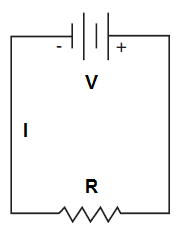A series circuit has only one path for current to flow. In a series circuit, current (I) is the same throughout the circuit and is equal to the total voltage (V) applied to the circuit divided by the total resistance (R) of the loads in the circuit. The sum of the voltage drops across each resistor in the circuit will equal the total voltage applied to the circuit.

###### Parallel Circuit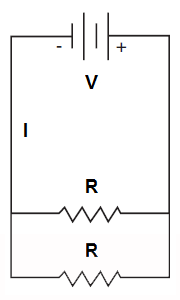In a parallel circuit, each load occupies a separate parallel path in the circuit and the input voltage is fully applied to each path. Unlike a series circuit where current (I) is the same at all points in the circuit, in a parallel circuit, voltage (V) is the same across each parallel branch of the circuit but current differs in each branch depending on the load (resistance) present.

###### Series-Parallel Circuits

Circuits are not limited to only series or only parallel configurations. Most circuits contain a mix of series and parallel segments. A good example is a household circuit breaker. Electrical outlets in each section of the house are wired in parallel with the circuit breaker for that section wired in series making it easy to cut off electricity to the parallel parts of the circuit when needed.

###### Battery Configurations

Batteries can be connected together in various combinations to increase their total voltage and/or total current. Connecting batteries in series combines their voltage while keeping their current the same, connecting batteries in parallel combines their current while keeping their voltage the same, and using a series-parallel configuration, half the batteries can be connected in series and half in parallel to combine both voltage and current.

### Electrical Systems

##### Types of Current
###### Direct Current (DC)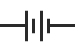Direct current flows in only one direction in a circuit, from the negative terminal of the voltage source to the positive. A common source of direct current (DC) is a battery.

###### Alternating Current (AC)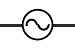In contrast to the constant one-way flow of direct current, alternating current changes direction many times each second. Electricity is delivered from power stations to customers as AC because it provides a more efficient way to transport electricity over long distances.

##### Electronic Components
###### Resistors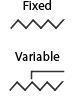Resistors are used to limit voltage and/or current in a circuit and can have a fixed or variable resistance. Variable resistors (often called potentiometers or rheostats) are used when dynamic control over the voltage/current in a circuit is needed, for example, in a light dimmer or volume control.

###### Fuses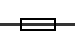Fuses are thin wires that melt when the current in a circuit exceeds a preset amount. They help prevent short circuits from damaging circuit components when an unusually large current is applied to the circuit, either through component failure or spikes in applied voltage.

###### Circuit Breakers

Like fuses, circuit breakers stop current flow once it reaches a certain amount. They have the advantage of being reusable (fuses must be replaced when "blown") but respond more slowly to current surges and are more expensive than fuses.

###### Capacitors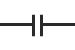Capacitors store electricity and are used in circuits as temporary batteries. Capacitors are charged by DC current (AC current passes through a capacitor) and that stored charge can later be dissipated into the circuit as needed. Capacitors are often used to maintain power within a system when it is disconnected from its primary power source or to smooth out or filter voltage within a circuit.

###### Diodes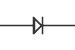A diode allows current to pass easily in one direction and blocks current in the other direction. Diodes are commonly used for rectification which is the conversion of alternating current (AC) into direct current (DC). Because a diode only allows current flow in one direction, it will pass either the upper or lower half of AC waves (half-wave rectification) creating pulsating DC. Multiple diodes can be connected together to utilize both halves of the AC signal in full-wave rectification.

###### TransistorsThe transistor is the foundation of modern electronic devices. It is made entirely from semiconductor material (making it a solid state device) and can serve many different functions in a circuit including acting as a switch, amplifier, or current regulator. A transistor works by allowing a small amount of current applied at the base to control general current flow from collector to emitter through the transistor.

###### Integrated Circuits

Circuits containing transistors are packaged into integrated circuit chips that allow encapsulating complex circuit designs (CPU, memory, I/O) for easier integration into electronic devices and machines.

###### Thermocouples

A thermocouple is a temperature sensor that consists of two wires made from different conductors. The junction of these two wires produces a voltage based on the temperature difference between them.

##### Magnetism
###### Magnetic Fields

A moving electric current produces a magnetic field proportional to the amount of current flow. This magnetic field can be made stronger by winding the wire into a coil and further enhanced if done around an iron containing (ferrous) core.

###### Inductors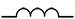An inductor is coiled wire that stores electric energy in the form of magnetic energy and resists changes in the electric current flowing through it. If current is increasing, the inductor produces a voltage that slows the increase and, if current is decreasing, the magnetic energy in the coil opposes the decrease to keep the current flowing longer. In contrast to capacitors, inductors allow DC to pass easily but resist the flow of AC.

###### Transformers

A transformer utilizes an inductor to increase or decrease the voltage in a circuit. AC flowing in a coil wrapped around an iron core magnetizes the core causing it to produce a magnetic field. This magnetic field generates a voltage in a nearby coil of wire and, depending on the number of turns in the wire of the primary (source) and secondary coils and their proximity, voltage is induced in the secondary coil.

# Mechanical Comprehension

• 94 Questions
• 34 Problems
• 100 Flash Cards

### Fundamentals of Mechanics

##### Principles
###### Mechanics

Mechanics deals with motion and the forces that produce motion.

###### Mass

Mass is a measure of the amount of matter in an object. In general, larger objects have larger mass than smaller objects but mass ultimately depends on how compact (dense) a substance is.

###### Inertia

The more mass a substance has the more force is required to move it or to change its direction. This resistance to changes in direction is known as inertia.

###### Net Force

In mechanics, multiple forces are often acting on a particular object and, taken together, produce the net force acting on that object. Like force, net force is a vector quantity in that it has magnitude and direction.

###### Newton's Second Law of Motion

Newton's Second Law of Motion states that "The acceleration of an object as produced by a net force is directly proportional to the magnitude of the net force, in the same direction as the net force, and inversely proportional to the mass of the object." This Law describes the linear relationship between mass and acceleration when it comes to force and leads to the formula F = ma or force equals mass multiplied by rate of acceleration.

###### Universal Gravitation

Newton's Law of Univeral Gravitation defines the general formula for the attraction of gravity between two objects: $$\vec{F_{g}} = { Gm_{1}m_{2} \over r^2}$$ . In the specific case of an object falling toward Earth, the acceleration due to gravity (g) is approximately 9.8 m/s2.

###### Weight

Mass is an intrinsic property of matter and does not vary. Weight is the force exerted on the mass of an object due to gravity and a specific case of Newton's Second Law of Motion. Replace force with weight and acceleration with acceleration due to gravity on Earth (g) and the result is the formula for weight: W = mg or, substituting for g, weight equals mass multiplied by 9.8 m/s2.

###### Boyle's Law

Boyle's law states that "for a fixed amount of an ideal gas kept at a fixed temperature, pressure and volume are inversely proportional". Expressed as a formula, that's $$\frac{P_1}{P_2} = \frac{V_2}{V_1}$$

##### Friction
###### Kinetic Friction

Friction resists movement. Kinetic (also called sliding or dynamic) friction resists movement in a direction opposite to the movement. Because it opposes movement, kinetic friction will eventually bring an object to a stop. An example is a rock that's sliding across ice.

###### Static Friction

Static friction is friction between two or more solid objects that are not moving relative to each other. An example is the friction that prevents a box on a sloped surface from sliding farther down the surface.

###### Coefficient of Friction

Coefficient of friction (μ) represents how much two materials resist sliding across each other. Smooth surfaces like ice have low coefficients of friction while rough surfaces like concrete have high μ.

###### Normal Force

Normal force (FN) represents the force a surface exerts when an object presses against it.

###### Force of Friction

The formula for force of friction (Ff) is the same whether kinetic or static friction applies: Ff = μFN. To distinguish between kinetic and static friction, μk and μs are often used in place of μ.

###### Kinetic vs. Static Friction

For any given surface, the coefficient of static friction is higher than the coefficient of kinetic friction. More force is required to initally get an object moving than is required to keep it moving. Additionally, static friction only arises in response to an attempt to move an object (overcome the normal force between it and the surface).

###### Normal Force vs. Weight

Normal force arises on a flat horizontal surface in response to an object's weight pressing it down. Consequently, normal force is generally equal to the object's weight.

##### Other Forces
###### Drag

Drag is friction that opposes movement through a fluid like liquid or air. The amount of drag depends on the shape and speed of the object with slower objects experiencing less drag than faster objects and more aerodynamic objects experiencing less drag than those with a large leading surface area.

###### Tension

Tension is a force that stretches or elongates something. When a cable or rope is used to pull an object, for example, it stretches internally as it accepts the weight that it's moving. Although tension is often treated as applying equally to all parts of a material, it's greater at the places where the material is under the most stress.

###### Hydraulic Pressure

Hydraulics is the transmission of force through the use of liquids. Liquids are especially suited for transferring force in complex machines because they compress very little and can occupy very small spaces. Hydraulic pressure is calculated by dividing force by the area over which it is applied: P = F/A where F is force in pounds, A is area in square inches, and the resulting pressure is in pounds per square inch (psi).

###### Pascal's Law

Pascal's law states that a pressure change occurring anywhere in a confined incompressible fluid is transmitted throughout the fluid such that the same change occurs everywhere. For a hydraulic system, this means that a pressure applied to the input of the system will increase the pressure everywhere in the system.

###### Torque

Torque measures force applied during rotation: τ = rF. Torque (τ, the Greek letter tau) = the radius of the lever arm (r) multiplied by the force (F) applied. Radius is measured from the center of rotation or fulcrum to the point at which the perpendicular force is being applied. The resulting unit for torque is newton-meter (N-m) or foot-pound (ft-lb).

###### Principle of Moments

When a system is stable or balanced (equilibrium) all forces acting on the system cancel each other out. In the case of torque, equilibrium means that the sum of the anticlockwise moments about a center of rotation equal the sum of the clockwise moments.

##### Energy, Work, & Power
###### Joules

The Joule (J) is the standard unit of energy and has the unit $${kg \times m^2} \over s^2$$.

###### Kinetic Energy

Kinetic energy is the energy of movement and is a function of the mass of an object and its speed: $$KE = {1 \over 2}mv^2$$ where m is mass in kilograms, v is speed in meters per second, and KE is in joules. The most impactful quantity to kinetic energy is velocity as an increase in mass increases KE linearly while an increase in speed increases KE exponentially.

###### Potential Energy

Potential energy is the energy of an object by virtue of its position relative to other objects. It is energy that has the potential to be converted into kinetic energy.

###### Gravitational Potential Energy

Gravitational potential energy is energy by virtue of gravity. The higher an object is raised above a surface the greater the distance it must fall to reach that surface and the more velocity it will build as it falls. For gravitational potential energy, PE = mgh where m is mass (kilograms), h is height (meters), and g is acceleration due to gravity which is a constant (9.8 m/s2).

###### Conservation of Mechanical Energy

As an object falls, its potential energy is converted into kinetic energy. The principle of conservation of mechanical energy states that, as long as no other forces are applied, total mechanical energy (PE + KE) of the object will remain constant at all points in its descent.

###### Work

Work is accomplished when force is applied to an object: W = Fd where F is force in newtons (N) and d is distance in meters (m). Thus, the more force that must be applied to move an object, the more work is done and the farther an object is moved by exerting force, the more work is done.

###### Work-Energy Theorem

The work-energy theorem states that the work done by the sum of all forces acting on a particle equals the change in the kinetic energy of the particle. Simply put, work imparts kinetic energy to the matter upon which the work is being done.

###### Power

Power is the rate at which work is done, P = w/t, or work per unit time. The watt (W) is the unit for power and is equal to 1 joule (or newton-meter) per second. Horsepower (hp) is another familiar unit of power used primarily for rating internal combustion engines. A 1 hp machine does 550 ft⋅lb of work in 1 second and 1 hp equals 746 watts.

### Machines

##### Simple Machines
###### Types of Simple Machines

The six types of simple machines are the lever, wheel and axle, pulley, inclined plane, wedge, and screw.

Mechanical advantage is a measure of the force amplification achieved by using a tool, mechanical device or machine system. Such a device utilizes input force and trades off forces against movement to amplify and/or change its direction.

###### Efficiency

The efficiency of a machine describes how much of the power put into the machine is turned into movement or force. A 100% efficient machine would turn all of the input power into output movement or force. However, no machine is 100% efficient due to friction, heat, wear and other imperfections that consume input power without delivering any output.

###### Inclined PlaneAn inclined plane is a simple machine that reduces the force needed to raise an object to a certain height. Work equals force x distance and, by increasing the distance that the object travels, an inclined plane reduces the force necessary to raise it to a particular height. In this case, the mechanical advantage is to make the task easier. An example of an inclined plane is a ramp.

###### Wedge

The wedge is a moving inclined plane that is used to lift, hold, or break apart an object. A wedge converts force applied to its blunt end into force perpendicular to its inclined surface. In contrast to a stationary plane where force is applied to the object being moved, with a wedge the object is stationary and the force is being applied to the plane. Examples of a wedge include knives and chisels.

###### First-Class Lever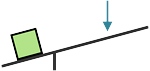A first-class lever is used to increase force or distance while changing the direction of the force. The lever pivots on a fulcrum and, when a force is applied to the lever at one side of the fulcrum, the other end moves in the opposite direction. The position of the fulcrum also defines the mechanical advantage of the lever. If the fulcrum is closer to the force being applied, the load can be moved a greater distance at the expense of requiring a greater input force. If the fulcrum is closer to the load, less force is required but the force must be applied over a longer distance. An example of a first-class lever is a seesaw / teeter-totter.

###### Second-Class Lever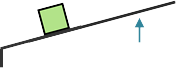A second-class lever is used to increase force on an object in the same direction as the force is applied. This lever requires a smaller force to lift a larger load but the force must be applied over a greater distance. The fulcrum is placed at one end of the lever and mechanical advantage increases as the object being lifted is moved closer to the fulcrum or the length of the lever is increased. An example of a second-class lever is a wheelbarrow.

###### Third-Class Lever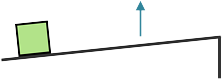A third-class lever is used to increase distance traveled by an object in the same direction as the force applied. The fulcrum is at one end of the lever, the object at the other, and the force is applied between them. This lever does not impart a mechanical advantage as the effort force must be greater than the load but does impart extra speed to the load. Examples of third-class levers are shovels and tweezers.

###### Fixed Pulley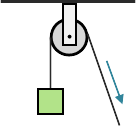A fixed pulley is used to change the direction of a force and does not multiply the force applied. As such, it has a mechanical advantage of one. The benefit of a fixed pulley is that it can allow the force to be applied at a more convenient angle, for example, pulling downward or horizontally to lift an object instead of upward.

###### Block and Tackle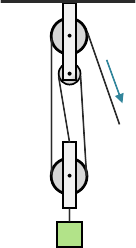Two or more pulleys used together constitute a block and tackle which, unlike a fixed pulley, does impart mechanical advantage as a function of the number of pulleys that make up the arrangement. So, for example, a block and tackle with three pulleys would have a mechanical advantage of three.

###### Wheel and Axle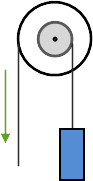A wheel and axle uses two different diameter wheels mounted to a connecting axle. Force is applied to the larger wheel and large movements of this wheel result in small movements in the smaller wheel. Because a larger movement distance is being translated to a smaller distance, force is increased with a mechanical advantage equal to the ratio of the diameters of the wheels. An example of a wheel and axle is the steering wheel of a car.

###### Screw

A screw is an inclined plane wrapped in ridges (threads) around a cylinder. The distance between these ridges defines the pitch of the screw and this distance is how far the screw advances when it is turned once. The mechanical advantage of a screw is its circumference divided by the pitch.

##### Gears
###### Gear Trains

Connected gears of different numbers of teeth are used together to change the rotational speed and torque of the input force. If the smaller gear drives the larger gear, the speed of rotation will be reduced and the torque will increase. If the larger gear drives the smaller gear, the speed of rotation will increase and the torque will be reduced.

###### Gear Ratio

The mechanical advantage (amount of change in speed or torque) of connected gears is proportional to the number of teeth each gear has. Called gear ratio, it's the ratio of the number of teeth on the larger gear to the number of teeth on the smaller gear. For example, a gear with 12 teeth connected to a gear with 9 teeth would have a gear ratio of 4:3.

### Applications

##### General
###### Force Lines of Action

Collinear forces act along the same line of action, concurrent forces pass through a common point and coplanar forces act in a common plane.

###### Bridge Forms

The six basic bridge forms are beam, truss, arch, cantilever, cable, and suspension.

###### Modulus of Elasticity

The modulus of elasticity measures how much a material or structure will deflect under stress. Stretch modulus is longitudinal stretch (like stretching raw bread dough), shear modulus is longitudinal deflection (like the horizontal displacement of a stack of magzines when a heavy object is placed upon them), and bulk modulus is compression of volume (like the compression of a loaf of bread under a heavy can at the bottom of a grocery bag).

###### Ceramics

Ceramics are mixtures of metallic and nonmetallic elements that withstand exteme thermal, chemical, and pressure environments. They have a high melting point, low corrosive action, and are chemically stable. Examples include rock, sand, clay, glass, brick, and porcelain.

###### Specific Gravity

Specific gravity is the ratio of the density of equal volumes of a substance and water and is measured by a hyrdometer.

Dead load is the weight of the building and materials, live load is additional weight due to occupancy or use, snow load is the weight of accumulated snow on a structure and wind load is the force of wind pressures against structure surfaces.

A concentrated load acts on a relatively small area of a structure, a static uniformly distributed load doesn't create specific stress points or vary with time, a dynamic load varies with time or affects a structure that experiences a high degree of movement, an impact load is sudden and for a relatively short duration and a non-uniformly distributed load creates different stresses at different locations on a structure.

# Shop Information

• 62 Questions
• 68 Flash Cards

### Hand Tools

##### Measuring Tools
###### Rulers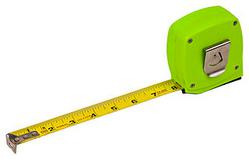Rulers are used to both measure distance and to draw straight lines. Tape measures are flexible rulers made from cloth or metal and are useful for measuring longer distances and in tighter spaces. Both rulers and tape measures provide similar accuracy, commonly down to $${1 \over 16}$$ or $${1 \over 32}$$ inch. A steel framing square is made up of a shorter ruler (tongue) and a longer ruler (blade) that meet at a 90° (right) angle. A framing square is often used by carpenters when framing stairs and roofs.

###### Micrometers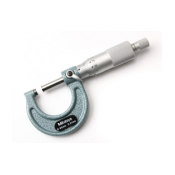Micrometers provide accuracy to the thousandths of an inch and come in outside and inside varieties. An outside micrometer is used to measure outside thickness, such as the diameter of a bolt, while an inside micrometer is used to measure the inside dimension of an object, such as the diameter of the hole of a nut or the width of a channel.

###### Calipers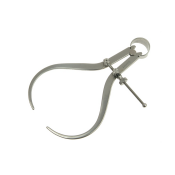Calipers are similar to micrometers in shape but instead of measuring distances, calipers are used to transfer distances between objects. An outside caliper is used to transfer outside dimensions while an inside caliper is used to transfer inside distances. A vernier caliper is an extremely precise caliper (down to $${1 \over 1000}$$ inch) that allows measuring / transferring either inside or outside dimensions.

###### Levels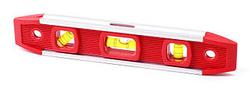Levels utlize a fluid-filled tube containing a bubble that is centered when the surface is horizontal (level) or vertical (plumb) along the direction of the tube. While a standard level can measure along a single dimension, a bullseye level is circular and can indicate the levelness of a two dimensional plane.

##### Striking Tools & Fasteners
###### Hammers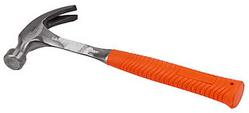The most common striking tool is the hammer and the most common variety of hammer is the claw hammer. The claw hammer has two ends, one to drive nails and one to remove nails. Ball-peen hammers replace the claw with a rounded end that's used to round off the edges of metal pins and make gaskets. A sledge hammer is a two-handed long-handled hammer with a large steel head used for heavy duty jobs.

###### Mallets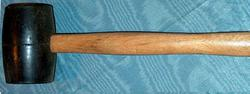A mallet is a hammer with a relatively large head, often made of rubber or wood. Both the size and material of the mallet head help prevent damage when striking more delicate surfaces.

###### Fasteners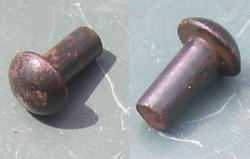A nail is a short pin-shaped shaft of steel that's typically used to fasten pieces of wood together. It has a flat head on one end and a point on the other. A rivet consists of a cylindrical shaft with a head on one end and a tail on the other. When the rivet is installed, the tail is expanded and reshaped to form another head, creating a dumbell shape that will hold two surfaces together semi-permanently.

##### Chisels & Punches
###### Chisels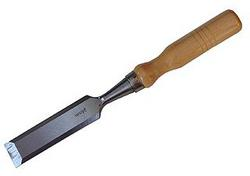A chisel has a long sharp edge and is used, often in conjunction with a hammer, for cutting. In woodworking, chisels are used to remove large sections of wood to create the initial shape of a design. In metalworking, chisels are used to remove waste metal when a smooth finish is not required.

###### Punches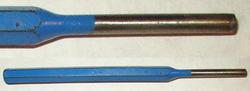A punch is narrow and is used to drive objects like nails (pin punch) or for making guide marks for drilling (center punch) or patterns in wood or metal.

##### Turning Tools & Fasteners
###### Screwdrivers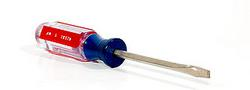Screwdrivers come in many different handle, shaft, and tip configurations for use in a wide variety of applications. Screwdrivers are classified by their tip which is shaped to fit a corresponding screw head. Common tips are slotted (flat) and Phillips (x-shaped).

###### Wrenches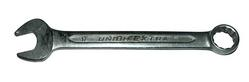Wrenches are used to provide grip and mechanical advantage by applying torque to turn objects (or to keep them from turning). The longer the wrench, the more torque that can be applied. Wrench ends are available in two primary types, open-end and box-end. A box-end wrench encloses the bolt head and is useful when more torque is needed or to maintain contact in difficult to reach locations. An open-end wrench is designed for speedily loosening easier to reach fasteners. Wrenches that feature one open and one box end are called combination wrenches and adjustable wrenches feature an open end with an adjustable width.

###### Ratchets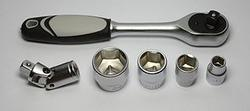A ratchet (or socket wrench) is a wrench that applies torque in only one direction with a handle that can be moved back and forth without losing contact with the fastener. A ratchet uses variable attachments called sockets which come in a variety of drive sizes based on the size of the opening that attaches to the ratchet. Sockets with the same drive size will vary in the shape (six-point, twelve-point) and size of the nut opening that attaches to the fastener being tightened or loosened. Smaller point sized sockets are stronger and can apply greater torque while larger point sizes allow easier alignment.

###### Fasteners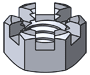Wrenches are used with threaded fasteners like bolts and nuts. A bolt has external threads while a nut has internal threads and this thread pattern combination allows them to lock together and act as fasteners. Nuts come in a variety of configurations including wing nuts which provide appendages that allow tightening and loosening by hand, slotted nuts that use a cotter pin to lock the nut in place and prevent it from loosening, and lock nuts that also prevent loosening via nylon in their threads. Threads are identified by pitch which is the number of threads per inch.

##### Gripping & Pincing Tools
###### Pliers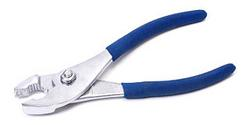Pliers are designed to provide a mechanical advantage, allowing the force of the hand's grip to be amplified and focused with precision. Pliers also allow finer control over objects that are too small to be manipulated by the fingers alone. The standard configuration is combination pliers which provide a fixed maximum jaw width. Other styles include adjustable joint pliers that allow selecting jaw width, needle nose pliers for holding small objects in tight spaces and locking pliers that will lock in place to hold or clamp objects together.

###### Pincers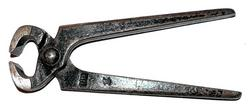Pincers provide a mechanical advantage that's used to cut, pinch or pull an object. The force applied to pincers is concentrated to a point or to an edge of the tool which allows pincers to be brought very close to a surface. Pincers are typically used for removing objects from a material to which they've previously been applied, for example, to pull nails from wood.

###### Clamps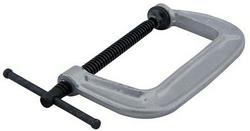A clamp applies pressure to prevent two objects from moving relative to each other, for example, to keep to pieces of wood together while the glue between the dries. A clamp is commonly made of metal and shaped like the letter C with a perpendicular flat-edged plate providing variable pressure between it and the top of the C as a screw to which it is attached is tightened and released.

###### Vises

A vise is a clamp that is anchored to a work station and designed to hold material in place while it is being operated upon.

##### Soldering & Welding
###### Soldering

Soldering is a low-temperature process by which two or more items (typically metal) are joined together by melting a filler metal (solder) into the joint. An electrically powered soldering iron or soldering gun is used to melt the solder which is an alloy of lead and tin that has a melting point below the melting point of the items being joined. A chemical cleaning agent called flux is also used to clean the surfaces before soldering.

###### Welding

Welding is a high-temperature process that involves melting the base metals in the objects to be joined to fuse them together. A filler metal is used to provide additional material to make up a joint that, depending on the weld type, can be stronger than the base materials alone. Oxyacetylene welding is a welding process that uses a torch fueled with oxygen and acetylene gases. Electric-arc welding utlizes electric current in a safer welding process (it doesn't involve burning explosive gases) that enables a wide variety of specialized applications like stick, MIG (metal inert gas), and TIG (tungsten inert gas) welding.

##### Saws
###### Wood Saws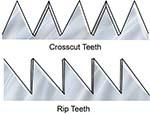Wood saws are categorized by their teeth shape and the number of teeth per inch (TPI). The higher the TPI of a saw the finer the cut it will make. Crosscut saws utilize knife-shaped teeth that cut across the grain of the wood while rip saws cut with the grain using chisel-shaped teeth that rip the wood cells apart as the cut is made. The kerf (slot) made by by a crosscut saw is much smoother than that made by a rip saw but a rip saw cuts much faster. Coping saws are a type of bow saw used to make detailed often curving cuts using replaceable blades with fine small teeth.

###### Hacksaw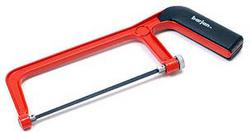A hacksaw has replaceable blades and is used to cut metal. The blade type is chosen based on the material that is to be cut. Blades with larger numbers of teeth per inch are more appropriate for cutting thinner materials.

###### Miter Box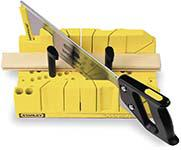A miter box utlizes a back saw (a fine toothed saw with a rigid strip of steel opposite its blade edge) to make cuts in wood at a specified angle.

##### Drills
###### Drilling

Drilling is the process of making small holes in wood or metal while boring is the process of making larger holes.

###### Drill Bits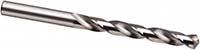Drill bits remove material to create holes. They come in a variety of sizes, maxing out at ¼" for woodworking and ½" for metalworking. The majority of drill bits are right-handed which means they cut while rotating in a clockwise direction.

###### Chucks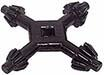Electric drills utilize a chuck to hold the drill bit. A chuck's size indicates the largest diameter drill bit that will fit. A chuck is tightened and loosened using a chuck key.

##### Finishing Tools
###### Planes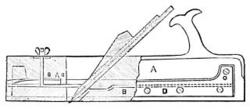In wooodworking, a plane is used to shave off a small amount of material to smooth a surface or make it fit properly. A jack plane is a general purpose plane that contains an adjustable depth blade set at an angle with a handle and knob to allow gripping when sliding the plane across wood.

###### Chisels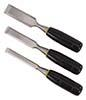Wood chisels are used to shape or smooth wood surfaces. They come in a variety of widths and can be used with hand power or tapped with a mallet when deeper cuts need to be made.

###### Files

Files consist of diagonal rows of fine teeth and are used for fine finishing work to smooth, polish, or shape objects. Rasps are files with larger teeth that are used for coarse finishing work. Both files and rasps are designed to be inserted into detachable handles.

###### Routers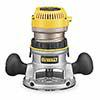A router is a tool that a worker uses to rout (hollow out), shape, or contour an area in relatively hard material like wood or plastic.